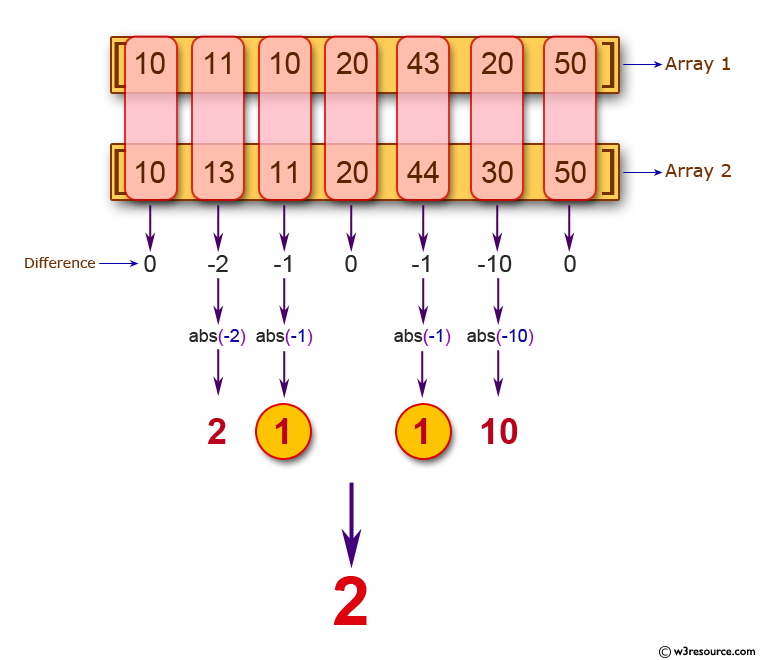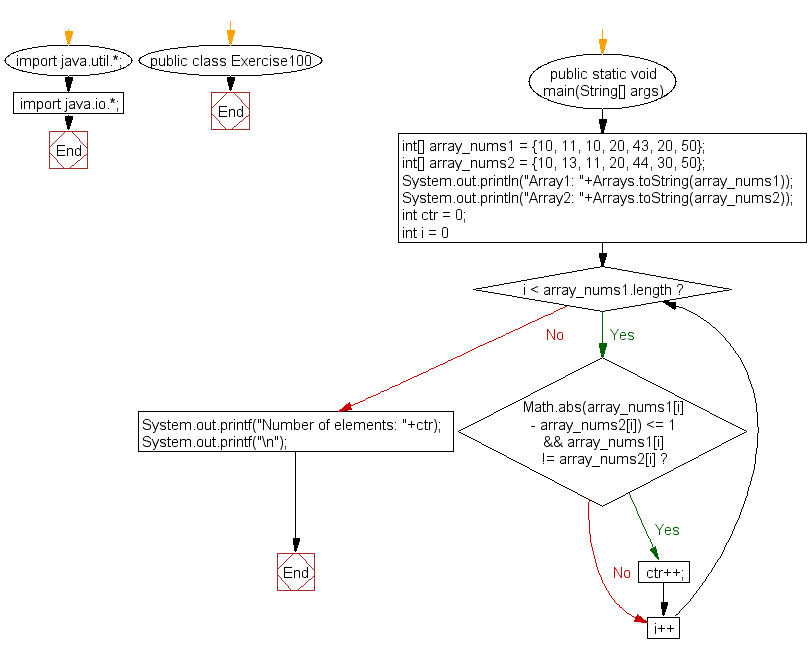﻿ Java: Count 2 elements of 2 arrays with same length# Java Exercises: Count the two elements of two given arrays of integers with same length, differ by 1 or less

## Java Basic: Exercise-100 with Solution

Write a Java program to count the two elements of two given arrays of integers with same length, differ by 1 or less.

Pictorial Presentation:Sample Solution:

Java Code:

``````import java.util.*;
import java.io.*;
public class Exercise100 {
public static void main(String[] args)
{
int[] array_nums1 = {10, 11, 10, 20, 43, 20, 50};
int[] array_nums2 = {10, 13, 11, 20, 44, 30, 50};
System.out.println("Array1: "+Arrays.toString(array_nums1));
System.out.println("Array2: "+Arrays.toString(array_nums2));
int ctr = 0;

for(int i = 0; i < array_nums1.length; i++) {
if(Math.abs(array_nums1[i] - array_nums2[i]) <= 1 && array_nums1[i] != array_nums2[i])
ctr++;
}

System.out.printf("Number of elements: "+ctr);
System.out.printf("\n");
}
}
```
```

Sample Output:

```Array1: [10, 11, 10, 20, 43, 20, 50]
Array2: [10, 13, 11, 20, 44, 30, 50]
Number of elements: 2
```

Flowchart:Java Code Editor:

What is the difficulty level of this exercise?

Test your Programming skills with w3resource's quiz.

﻿

## Java: Tips of the Day

countOccurrences

Counts the occurrences of a value in an array.

Use Arrays.stream().filter().count() to count total number of values that equals the specified value.

```public static long countOccurrences(int[] numbers, int value) {
return Arrays.stream(numbers)
.filter(number -> number == value)
.count();
}
```

Ref: https://bit.ly/3kCAgLb